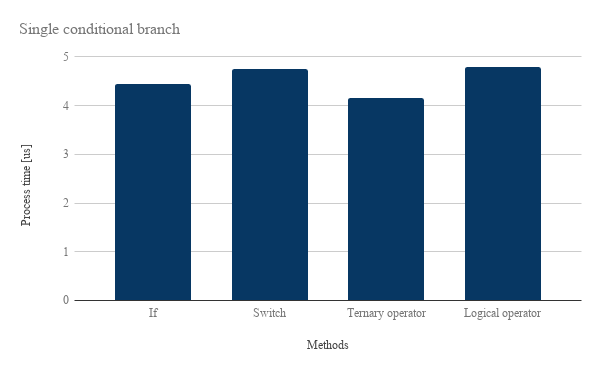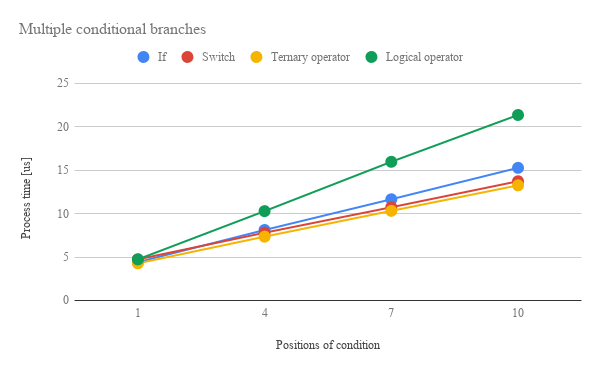Gists

Kanshi Tanaike

# Introduction

Please be careful! This result can be only used for Google Apps Script.

There are a limit executing time for Google Apps Script (GAS). That is 6 minutes.1 So users always have to pay attention to reducing the process cost of the scripts. Especially, it is very important to know the process cost for the array processing, because the array processing is often used for spreadsheet and Google APIs. Recently, I have already published some reports about the process cost using GAS.2-6 From these reports, it has found that GAS shows much different process cost from other languages. So it is important to investigate the process cost for various scenes. In this report, the process cost of “conditional branch” using GAS has been investigated.

Here, the following 4 methods for the conditional branch were used. And as the sample situation, a single condition branch and multiple condition branches (10 branches) are used for measuring the process cost. In this report, for example, a single conditional branch is if (value === "value") {r = "true"} else {r = "false"}.

1. If
2. Switch
3. Ternary operator
4. Logical operator

As the result, it was found that the cost of “Ternary operator” was the lowest of all methods and conditions. Namely, it was the fastest of all. About 2nd rank, the interesting results were obtained. For the single conditional branch, 2nd one was “If”. But for the multiple conditional branches, “Switch” was the 2nd one. This indicates that “If” and “Switch” are suitable for the single and multiple conditional branches, respectively. “Logical operator” was the lowest rank for the single and multiple conditional branches.

# Experimental procedure

In the experiment, the sample scripts for GAS were used.Appendix For 4 methods of “If”, “Switch”, “Ternary operator” and “Logical operator”, 2 conditions were used for measuring the process cost. Namely, the process times of them were measured. Those were the single conditional branch and the multiple conditional branches (10 branches). By the way, at GAS, the processing time is not stable as you know. So for each method, the average values which measured 1,000,000 times were used as the process time (process cost). Furthermore, the average value for more than 100 times measurements was used for each data point which is shown by figures. At this time, the fluctuation of the average values was less than 1 %. I worry that each detailed-data point at my environment might be different from that at other user’s environment. But I think that the trend of this result can be used.

# ResultsFig 1. Processing time (process cost) for single conditional branch.

Figure 1 shows the processing time (process cost) for single conditional branch. Since the absolute process time is microsecond order, it is considered that the differences of each method is a little. But when such conditional branch is used for many places in the script, the process cost will get to be able to be ignored. From Fig. 1, in the case of the single conditional branch, the process cost becomes higher in order of “Ternary operator”, “If”, “Switch” and “Logical operator”. For the single conditional branch, using Fig. 1, the quantitative differences of these methods are as follows.

• Cost of “If” is 8 % larger than that of “Ternary operator”.
• Cost of “Switch” is 15 % larger than that of “Ternary operator”.
• Cost of “Logical operator” is 16 % larger than that of “Ternary operator”.
• When “If” is used for the single conditional branches, it can reduce the cost of 6 % for “Switch”.Fig 2. Processing time (process cost) for multiple conditional branches (10 branches). The solid lines with the color of blue, red, orange and green mean the result from “If”, “Switch”, “Ternary operator” and “Logical operator”, respectively. Horizontal axis means the process time of the position of branch when true is obtained. For example, when the condition is true at 7th branch, the position is 7.

Figure 2 shows the processing time (process cost) for multiple conditional branches (10 branches). The solid lines with the color of blue, red, orange and green mean the result from “If”, “Switch”, “Ternary operator” and “Logical operator”, respectively. Horizontal axis means the process time of the position of branch when true is obtained. For example, when the condition is true at 7th branch, the position is 7. From Fig. 2, the process cost linearly increases with the increase in the position of condition. For one method, it is found that the comparison of the variable is carried out every branch and each cost is the same. This means that the cost can be reduced by put the condition with the high possibility of “true” to the fore. In the case of multiple conditional branches (more than 2 branches), the process cost becomes higher in order of “Ternary operator”, “Switch”, “If” and “Logical operator”. For the multiple conditional branches, using Fig. 2, the quantitative differences of these methods are as follows.

• Cost of “If” is 25 % larger than that of “Ternary operator”.
• Cost of “Switch” is 2 % larger than that of “Ternary operator”.
• Cost of “Logical operator” is 96 % larger than that of “Ternary operator”.
• When “Switch” is used for the multiple conditional branches, it can reduce the cost of 25 % for “If”.

When it looks carefully at Figs. 1 and Fig. 2, it is found that the process costs of “If” and “Switch” invert between Fig. 1 and Fig. 2. When the slope of Fig. 2 is calculated, the difference can be found well.Fig 3. Slope for each method calculated from Fig. 2.

Figure 3 shows the the slope for each method calculated from Fig. 2. So the unit is microseconds/position. From Fig. 3, it is found that at the case of multiple conditional branches, “Switch” is more suitable than “If”. So from Figs. 1, 2 and 3, It is considered that this indicates that “If” and “Switch” are suitable for the single conditional branch and the multiple conditional branches, respectively. And it is found that “Ternary operator” can be used for both conditional branches with the low cost. On the other hand, it is considered that “Logical operator” is not suitable for the general use, because of the high cost and low readability.

# Summary

In this report, the process cost of “conditional branch” using GAS has been investigated. 4 methods of “If”, “Switch”, “Ternary operator” and “Logical operator” were used for this report. The following results were obtained.

1. It was found that the cost of “Ternary operator” was the lowest of all methods and conditions.
2. For the single conditional branch, 2nd one was “If”. But for the multiple conditional branches, “Switch” was the 2nd one. This indicates that “If” and “Switch” are suitable for the single and multiple conditional branches (more than 2 branches), respectively.
3. In the case of the multiple conditional branches, the process cost can be reduced by put the condition with the high possibility of “true” to the fore.
4. “Logical operator” was the lowest rank for the single and multiple conditional branches. It is considered that “Logical operator” is not suitable for the general use, because of the high cost and low readability.

As a note, I have to describe that this is the result for Google Apps Script. For other languages, this result might be difference. And also, the process cost of this report might be modified by future update of Google.

## Appendix

### Scripts

#### Sample scripts for a single conditional branch.

// If
if (value === "value") {
r = "true";
} else {
r = "false";
}

// Switch
switch (value) {
case "value":
r = "true";
break;
default:
r = "false";
break;
}

// Ternary operator
r = value === "value" ? "true" : "false";

// Logical operator
r = value === "value" && "true" || "false";


#### Sample scripts for the multiple conditional branches (10 branches).

// If
if (value === "value1") {
r = "value1";
} else if (value === "value2") {
r = "value2";
} else if (value === "value3") {
r = "value3";
} else if (value === "value4") {
r = "value4";
} else if (value === "value5") {
r = "value5";
} else if (value === "value6") {
r = "value6";
} else if (value === "value7") {
r = "value7";
} else if (value === "value8") {
r = "value8";
} else if (value === "value9") {
r = "value9";
} else if (value === "value10") {
r = "value10";
} else {
r = "false";
}

// Switch
switch (value) {
case "value1":
r = "value1";
break;
case "value2":
r = "value2";
break;
case "value3":
r = "value3";
break;
case "value4":
r = "value4";
break;
case "value5":
r = "value5";
break;
case "value6":
r = "value6";
break;
case "value7":
r = "value7";
break;
case "value8":
r = "value8";
break;
case "value9":
r = "value9";
break;
case "value10":
r = "value10";
break;
default:
r = "false";
break;
}

// Ternary operator
r = value === "value1" ? "value1"
: value === "value2" ? "value2"
: value === "value3" ? "value3"
: value === "value4" ? "value4"
: value === "value5" ? "value5"
: value === "value6" ? "value6"
: value === "value7" ? "value7"
: value === "value8" ? "value8"
: value === "value9" ? "value9"
: value === "value10" ? "value10"
: "false";

// Logical operator
r = value === "value1" && "value1"
|| value === "value2" && "value2"
|| value === "value3" && "value3"
|| value === "value4" && "value4"
|| value === "value5" && "value5"
|| value === "value6" && "value6"
|| value === "value7" && "value7"
|| value === "value8" && "value8"
|| value === "value9" && "value9"
|| value === "value10" && "value10"
|| "false";